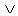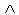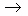Logic
edHelper subscribers - Create a new printable

Number of Keys
 Select the number of different printables: 1 key 2 keys 3 keys 4 keys 5 keys

Number of Pages (for each key)
 Select the number of pages: 1 page 2 pages 3 pages 4 pages

Include an answer key (answer keys will be at the end of the printable)
High School Geometry
High School Geometry
Math

 Name _____________________________Date ___________________
Logic
State the truth value of the following statements.

 1 If ~ pq is true, what conclusion can be drawn from the statement: p~ q
 2 If ~ pq is false, what conclusion can be drawn from the statement: pq
 3 If p~ q is false, what conclusion can be drawn from the statement:~ p~ q
 4 If ~ pq is false, what conclusion can be drawn from the statement:~ pq
 5 If p~ q is true, what conclusion can be drawn from the statement:(~ q~ p )(~ pq )
 6 If ~ p~ q is true, what conclusion can be drawn from the statement: q( p~ q )
 7 If ~ p~ q is true, what conclusion can be drawn from the statement:~(( q~ p )(~ pq ))
 8 If ~ p~ q is true, what conclusion can be drawn from the statement: p~ q
 9 If ~ pq is true, what conclusion can be drawn from the statement:~(~ pq )q
 10 If pq is false, what conclusion can be drawn from the statement:~ pq
 11 If ~ pq is false, what conclusion can be drawn from the statement:( q~ p )q
 12 If p~ q is true, what conclusion can be drawn from the statement:~(~ p~ q )
 13 If ~ pq is true, what conclusion can be drawn from the statement:~(( qp )( q~ p ))
 14 If ~ pq is true, what conclusion can be drawn from the statement: pq
 15 If ~ p~ q is false, what conclusion can be drawn from the statement:~(( qp )~(~ qp ))
 16 If p~ q is false, what conclusion can be drawn from the statement: p([~( pq )])

Sample
This is only a sample worksheet.

Logic
edHelper subscribers - Create a new printable

Number of Keys
 Select the number of different printables: 1 key 2 keys 3 keys 4 keys 5 keys

Number of Pages (for each key)
 Select the number of pages: 1 page 2 pages 3 pages 4 pages